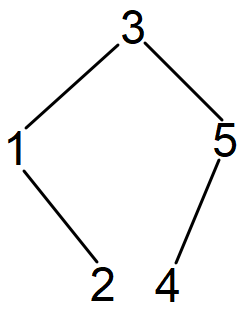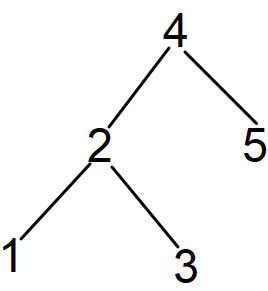# Maximum height of the binary search tree created from the given array

## Introduction:

We are given an array of ‘N’ integers. We need to construct two Binary Search Trees starting from both the left and right ends, and then return the maximum height of the Binary Search Tree among both of them.

Let us see a few examples:-

Input: [3, 1, 5, 2, 4]

Output: 2

Explanation: The following two trees will be formed from both the left and right ends.Both the Binary Search Trees have a height of 2. Hence, the maximum height is 2.

## Approach:

We will first implement a class for Tree. We will implement the Insert function so that we can add a node to the Binary Search Tree.

Using our tree class, we will construct both the Binary Search Trees from the left and right end and then find the height of both the trees and then return the maximum among them.

Refer to the below implementation of the above approach.

Time Complexity: The time complexity for the above approach is O(N), (where ‘N’ is the total number of nodes in the tree) because we are just constructing a tree that takes O(N) time and finding the depth of the tree also takes O(N) time.

Space Complexity: The space complexity for the above code is O(N) because we are constructing two trees of ‘N’ nodes.

## FAQs:

1. What is a Binary Search Tree?
• A tree which has utmost two children. All the nodes on the left of the current node must have a value less than the current node’s value whereas all the nodes on the right side of the current node must have a value greater than the current node’s value.
2. What is the time complexity for the approach used?
• The time complexity for the above approach is O(N) because we are just constructing a tree that takes O(N) time and finding the depth of the tree also takes O(N) time.

## Key Takeaways:

In this blog, we have covered the following things:

1. We first discussed the approach to solve this problem.
2. Then we saw how we implemented the approach discussed.

If you want to learn more about Binary Search Tree and want to practice some questions which require you to take your basic knowledge on Binary Search Tree a notch higher, then you can visit our Guided Path for Binary Search Tree

Until then, All the best for your future endeavors, and Keep Coding.